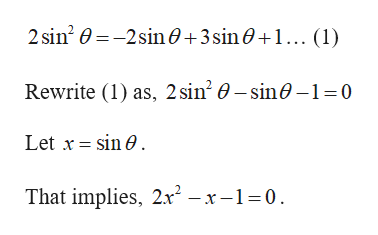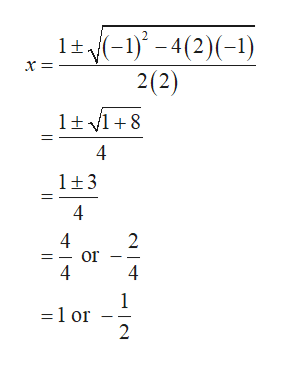Question
22 views

2sin2Θ = -2sinΘ + 3sinΘ + 1

check_circle

Step 1

The given trigonometric equation is,help_outlineImage Transcriptionclose2 sin? 0 =-2sin0+3sin0+1... (1) Rewrite (1) as, 2 sin? 0 – sin0 -1=0 Let x = sin 0. That implies, 2x – x –1=0. fullscreen
Step 2

Observe that the above equation is in the form of quadratic equation.

So consider a = 2, b = –1 and c = –1 and su...help_outlineImage Transcriptionclose1+(-1) – 4(2)(-1) 2(2) х%3 х- 1+1 +8 1+3 4 4 or 4 =1 or 4) fullscreen

### Want to see the full answer?

See Solution

#### Want to see this answer and more?

Solutions are written by subject experts who are available 24/7. Questions are typically answered within 1 hour.*

See Solution
*Response times may vary by subject and question.
Tagged in
MathCalculus

### Other